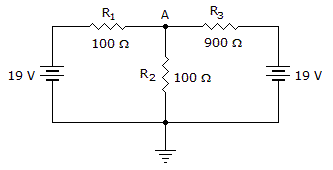# Electronics - Series-Parallel Circuits

### Exercise :: Series-Parallel Circuits - Filling the Blanks

1.

 A. the measured voltage is very high B. the measured voltage is very low C. the meter's resistance is at least 10 times greater than the resistance across which it is connected D. the meter's resistance is at least 10 times less than the resistance across which it is connected

Explanation:

No answer description available for this question. Let us discuss.

2.

A Thevenin equivalent circuit contains _____.

 A. a voltage source in series with a resistance B. a current source in series with a resistance C. resistors in a series-parallel configuration D. many voltage sources

Explanation:

No answer description available for this question. Let us discuss.

3.In the given circuit, the current flowing through R3 equals ______.

 A. 10 mA B. 21.11 mA C. 90 mA D. 100 mA

Explanation:

No answer description available for this question. Let us discuss.

4.

Series components in a series–parallel circuit may be in series with other _______________ components, or with other _______________ components.

 A. individual, combinations of B. series, parallel C. parallel, series D. shunt, parallel

Explanation:

No answer description available for this question. Let us discuss.

5.

If four 10 kresistors are in series with a single 20 kresistor and one of the parallel resistors shorts, the voltage across the other parallel resistors ________.

 A. increases B. decreases C. remains the same D. drops to zero